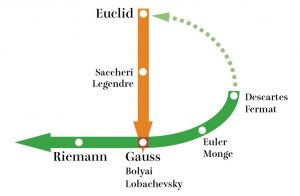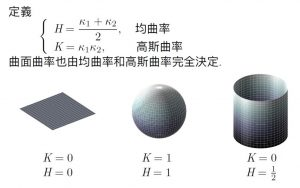# 从模函数到单值化定理 Ⅴ

Prologue:

…It is true that Mr. Fourier had the opinion that the principal purpose of mathematics was the benefit of the society and the explanation of phenomena of nature; but a philosopher like he should know that the sole purpose of science is the honor of the human mind, and under this title, a question about numbers is as valuable as a question about the system of the world…

——C. G. Jacobi, Letter to Legendre

$$f_{i}(z+1)=f_{i}(z)，f_{i}(z+\tau)=e^{-2k\pi iz}f_{i}(z)$$

$S$ 当然不能嵌入 $\mathbb{C}P^{1}$。取 $k=3$，利用上述的 $f_{i}$ 可完成 $S$ 到 $\mathbb{C}P^{2}$ 的嵌入。我们不再讨论技术性的细节，而是指出类似的想法可以推广到高维。高维复环面可以嵌入射影空间当且仅当其周期矩阵满足Frobenius关系。

Weierstrass提出了另一种构造椭圆函数的方法，即利用Weierstrass $\mathfrak{P}$函数。这方法简洁明了，被大多数现代课本采用。然而值得指出的是，theta函数处在数论、自守形式、函数论和数学物理的交叉点上，研究其性质有极高的附加价值。在第7章中有一个重要的例子：尖点形式$\Delta$。

Jacobi noted, as mathematics’ most fascinating property, that in it one and the same function controls both the presentations of a whole number as a sum of four squares and the real movement of a pendulum.

These discoveries of connections between heterogeneous mathematical objects can be compared with the discovery of the connection between electricity and magnetism in physics or with the discovery of the similarity between the east coast of America and the west coast of Africa in geology.

The emotional significance of such discoveries for teaching is difficult to overestimate. It is they who teach us to search and find such wonderful phenomena of harmony of the Universe.

Author: KKK

In this short note, we will give a simple proof of the Gauss-Bonnet theorem for a geodesic ball on a surface. The only prerequisite is the first variation formula and some knowledge of Jacobi field (second variation formula), in particular how its second derivative (or the second derivative of the Jacobian) is related to the curvature of the surface. This is different from most standard textbook proofs at the undergraduate level. (Of course, this is just a local version of the Gauss-Bonnet theorem and topology has not yet come into play.)

Let $M$ be a surface equipped with a Riemannian metric. We will fix a point $p$ in $M$ and from now on $B_r$ always denotes the geodesic ball of radius $r$ centered at $p$, and $\partial B_r$ its boundary, which is called the geodesic sphere. In geodesic polar coordinates, let the area element of $M$ be locally given by

$$\displaystyle \begin{array}{rl} \displaystyle dA=f(\theta, r) dr d\theta, =f_\theta(r) dr d\theta, \end{array}$$

where $f(\theta, r)$ is the Jacobian (with respect to polar coordinates). For our purpose it is more convenient to regard $f_\theta(r)$ as a one-parameter family of functions in the variable $r$. It is well-known that $f_\theta$ satisfies the Jacobi equation (here $’=\frac{d}{dr}$ )

$$\displaystyle \begin{array}{rl} \displaystyle {f_\theta}”(r)=-K(\theta, r) f_\theta(r),\quad f_\theta(0)=0,\quad {f_\theta}'(0)=1 \ \ \ \ \ (1)\end{array}$$

where $K=K(\theta, r)$ is the Gaussian curvature (in polar coordinates). Indeed, if we fix a geodesic polar coordinates, and $\gamma_\theta(t)$ is the arc-length parametrized geodesic with initial “direction” $\theta$ starting from $p$, then we can define a parallel orthonormal frame $e_1(t), e_2(t)=\gamma_\theta'(t)$ along $\gamma_\theta(t)$. Then $Y(t)=f_\theta(t)e_1(t)$ is a Jacobi field and so

$$\displaystyle \begin{array}{rl} \displaystyle Y”(t)={f_\theta}”(t) e_1 (t) =- R(Y(t), \gamma_\theta'(t))\gamma_\theta'(t) =& \displaystyle -K(\theta, t) Y(t)\\ =& \displaystyle -K(\theta, t) f_\theta (t) e_1(t). \end{array}$$

From this (1) follows.

The first variation formula says (here $s$ is the arclength parameter)

$$\displaystyle \begin{array}{rl} \displaystyle \frac{d}{dt} \left(\mathrm{Length}(\partial B_t)\right) =\frac{d}{dt} \left(\int_0^{2\pi}f_\theta( t) d\theta\right) =\int_{ \partial B_t}k_g ds =\int_0^{2\pi} k_g(\theta, t) f_\theta( t)d\theta. \end{array}$$

Here $k_g$ is the geodesic curvature of the geodesic circle $\partial B_t$. (Indeed, the differential version $\frac{f_\theta’}{f_\theta}=k_g$ is already true for the geodesic circle.) This implies

$$\displaystyle \begin{array}{rl} \displaystyle \int_{\partial B_t}k_g ds =\int_{0}^{2\pi} f_\theta'(t) d\theta. \end{array}$$

So by the fundamental theorem of calculus and (1), we have

$$\displaystyle \begin{array}{rl} \displaystyle \int_{\partial B_t}k_g ds =& \displaystyle \int_{0}^{2\pi}\left({f_\theta}'(0)+\int_0^t {f_\theta}”(r)dr\right)d\theta\\ =& \displaystyle \int_{0}^{2\pi}\left(1-\int_0^t K (\theta, r)f_\theta(r)dr\right)d\theta\\ =& \displaystyle 2 \pi-\int_{B_t} K dA. \end{array}$$

This is exactly the Gauss-Bonnet theorem (for a geodesic ball), which is usually written as

$$\displaystyle \begin{array}{rl} \displaystyle \int_{B_r}K dA+\int_{\partial B_r}k_g ds=2\pi. \end{array}$$

Prologue:

“转换原理”可以粗略地叙述如下：如果对“有界”函数叙述的较弱命题成立，则对“在 $$\mathbb{C}$$ 上有2个空隙值”的函数所叙述的较强命题也成立。

“转换原理”将其加强为著名的Picard小定理：

# 非欧几何是一个时代的结束？还是开始？

If you ask a mathematician “why?” the mathematician will give you a proof.
But when you ask a historian “why?” the historian will tell you a story.

## 设准 vs. 公理

Postulate = Special Notion vs. Common Notion = Axiom

We will raise this conjecture (the purport of which will hereafter be called the “Principle of Relativity”) to the status of a postulate, and also introduce another postulate, which is only apparently irreconcilable with the former, namely, that light is always propagated in empty space with a definite velocity:c which is independent of the state of motion of the emitting body. These two postulates suffice for the attainment of a simple and consistent theory of the electrodynamics of moving bodies based on Maxwell’s theory for stationary bodies. The introduction of a “luminiferous aether” will prove to be superfluous inasmuch as the view here to be developed will not require an “absolutely stationary space” provided with special properties, nor assign a velocity-vector to a point of the empty space in which electromagnetic processes take place.

## 数学真理的相关议题

“Snow is white” is true if snow is white.

## 爱因斯坦的反思！

Morris Kline在《数学：确定性的失落》中写道：「倘若数学命题是对现实(reality)的描述，它们就不是确定的(certain)；倘若它们是确定的，那它们就不是描述现实。……然而另一方面，可以确定的是，不论就数学整体或是单就几何而言，它们的存在都是我们想要得知实际物体的性质。

## 非欧几何的历史

15世纪文艺复兴绘画开始发展的「透视」和「无穷远点」等射影几何概念，不被想成「非」欧氏几何，而是关联于欧氏几何发展的领域。

1. 绝对欧氏空间的扬弃，把实际空间还给物理学，把欧氏几何想成一种可能的几何（最简单的）。
2. 非欧几何必须相应处理原来欧氏几何的几何概念：长度、角度、面积、体积等。## 高斯曲率是内禀的几何量## 测量高斯曲率

Gauss-Bonnett定理之原版：三角形内角和

$\theta_1 + \theta_2+ \theta_3=\iint_{\triangle} K\mathrm dA.$

## 康德的复仇

“苟日新，日日新，又日新”

Ahlfors《复分析》最精彩的部分在于提供了一个从拓扑角度看完全清晰而现代的Cauchy积分定理。他毫不掩饰自己的分析学家趣味，自得其乐地(当然同时也让读者受益无穷)讨论着函数的各种表示，函数空间内的收敛性，椭圆函数论以及超几何函数论。这些论题覆盖了经典函数论的绝大部分内容，又出之以现代观点，使得此书在数十年间一直保持着第一参考书的地位。这是3本书中我读得最早也最钟爱的一本，虽然我并不强求它是完美的：在不引入Riemann面的情况下引入层论是相当勉强的，除了让叙述稍显摩登之外没有什么意义。

Cartan的《解析函数论》则处于一种尴尬的境地。他试图把Weierstrass观点摆到图像的中心，但这里有一个天然的(？)的限制：从积分表示构造幂级数表示要比从幂级数表示构造积分表示自然得多。因而他不得不在两种观点间来回跳跃，远不如Ahlfors宏大而一致。当然他的牺牲也获得了某种回报：Weierstrass观点可以毫不费力地推广到多变元(Cauchy的大部分函数论定理也仍然正确；高维的困难之处本质上是几何的)。

Theorem. There does not exist a group whose commutator subgroup is isomorphic to $$S_4$$.

The relevant facts are that $$S_4$$ is a complete group(no outer automorphisms, trivial center) which is not perfect(that is, the commutator subgroup of $$S_4$$ is not $$S_4$$ itself). Any group which has these properties is never a commutator subgroup of anything. Here’s why.

Lemma. If $$K$$ is a complete group and $$K\lhd G$$, then $$G$$ is the direct product $$K\times H$$ of $$K$$ by its centralizer $$H=C_G(K)$$.

In other words, a complete group can be a normal subgroup only in the most trivial fashion: the large group is just a direct product of the normal group by something.

Proof of the lemma. Let $$H=C_G(K)$$ be the centralizer of $$K$$ in $$G$$, namely the set of all elements which commute with all elements of $$K$$. $$H$$ is a normal subgroup of $$G$$, and $$H\cap K=Z(K)=1$$ since $$K$$ has trivial center. Any element $$g\in G$$ induces an automorphisms $$\phi_g$$ of $$K$$ by conjugation: $$\phi_g(k)=g^{-1}kg$$. But $$K$$ has no outer automorphisms, so $$\phi_g$$ must equal some inner automorphism of $$K$$, that is, for some $$k\in K$$, $$\phi_g=\phi_k$$. Now conjugation by $$gk^{-1}$$ does nothing to $$K$$, so $$gk^{-1}=h\in H$$. In other words $$g=kh$$: every element of $$G$$ is expressible as product of an element of $$K$$ and an element of $$H$$. Since $$H$$ and $$K$$ commute, $$G$$ is the direct product of $$H$$ and $$K$$.            $$\Box$$

Proof. Now suppose that $$G$$ is some group such that $$K=G^\prime=[G,G]$$, the commutator subgroup, is such that $$K$$ is complete and non-perfect. By the lamma, $$G=K\times A$$ where $$A\cong G/K$$ is an abelian group. So any element of $$G$$ can be writeen as $$ka$$ with $$k\in K$$ and $$a\in A$$, and moreover, $$ka=ak$$ for any $$k\in K$$, $$a\in A$$.

Consider a commutator $$c=xyx^{-1}y^{-1}$$ in $$G$$. Write $$x=ka$$ and $$y=lb$$. Since $$K$$ and $$A$$ commute, $$c=aba^{-1}b^{-1}xyx^{-1}y^{-1}$$. Since $$A$$ is abelian, the first part vanishes and $$c=xyx^{-1}y^{-1}$$. So any commutator of $$G$$ lies in the commutator sungroup of $$K$$, and it follows that $$G^\prime=K^\prime$$. Since $$K^\prime\ne K$$, $$G^\prime\ne K$$, as well.

It remains to show that $$S_4$$ is complete and non-perfect.

(i) $$S_4$$ has trivial center: this is obvious. No permutation commutes with all other permutations.

(ii) $$S_4$$ has no outer automorphisms. This is true for all $$S_n$$ except $$n=2$$, $$6$$. It’s a standard result.

(iii) $$S_4$$ is not perfect. This is also ovious: for any two permutations $$\sigma$$, $$\tau\in S_n$$, the commutator $$\sigma^{-1}\tau^{-1}\sigma\tau$$ is an even permutation, so the commutator subgroup is contained in the alternating group $$A_n$$. In fact the commutator group eauals $$A_n$$, but we don’t need that here.

This completes the proof the theorem.                                  $$\Box$$

Remark. a simple modification: $$K^\prime\subset G^\prime$$ is clear, and the other direction follows since $$G/K^\prime=K/{K^\prime\times A}$$ is abelian.

Here is a proof not using those well-known facts about $$S_4$$. (though it’s easy to derive them with it)

Proof. $$S_4$$ has exactly $$4$$ Sylow  $$3$$-subgroups

$P_1=\langle(234)\rangle, P_2=\langle(134)\rangle, P_3=\langle(124)\rangle, P_4=\langle(123)\rangle,$

where $$P_i$$ is the only Sylow $$3$$-subgroup of the stabilizer of $$i$$ in $$S_4$$ for $$i=1$$, $$2$$, $$3$$, $$4$$. So $$\sigma P_i\sigma^{-1}=P_{\sigma(i)}$$ for all $$\sigma\in S_4$$ and $$i=1$$, $$2$$, $$3$$, $$4$$.

Assume $$G^\prime=S_4$$ and take $$g\in G$$. The conjugation with $$g$$ permutes $$P_1$$, $$P_2$$, $$P_3$$, $$P_4$$, so we find $$\rho\in G$$ with $$g P_ig^{-1}=P_{\rho(i)}=\rho P_i\rho^{-1}$$ for $$i=1$$, $$2$$, $$3$$, $$4$$. So $$h\colon \rho^{-1}g\in G$$ satisfies $$h P_ih^{-1}=P_i$$ for $$i=1$$, $$2$$, $$3$$, $$4$$.

Then for all $$\sigma\in S_4$$ we have also $$h^{-1}\sigma h\in S_4$$ and

\begin{equation} \begin{split}P_{h^{-1}\sigma h(i)}&=(h^{-1}\sigma h)P_i(h^{-1}\sigma h)^{-1}\\&=h^{-1}\sigma hP_ih^{-1}\sigma^{-1}h\\&=h^{-1}\sigma P_i\sigma^{-1}h\\&= h^{-1} P_{\sigma(i)}h=P_{\sigma(i)}.\end{split} \end{equation}

and therefore $$h^{-1}\sigma h(i)=\sigma(i)$$ for $$i=1$$, $$2$$, $$3$$, $$4$$.

This gives $$h\in C_G(S_4)$$ and $$g=\rho h\in S_4C_G(S_4)$$ for all $$g\in G$$. So $$G=S_4C_G(S_4)$$ and $$|G\colon A_4C_G(S_4)|\leqslant2$$. But then $$A_4C_G(S_4)\trianglelefteq G$$ with abelian factor, so $$S_4=G^\prime\leq A_4C_G(S_4)$$, and by Dedekind we get

$S_4=A_4C_G(S_4)\cap S_4=A_4(C_G(S_4)\cap S_4)=A_4Z(S_4)=A_4$

since $$Z(S_4)=1$$, as $$\sigma\in Z(S_4)$$ would give $$P_i=\sigma P_i\sigma^{-1}=P_{\sigma(i)}$$ and $$i=\sigma(i)$$ for $$i=1$$, $$2$$, $$3$$, $$4$$. Contradiction!

Darboux (14 August 1842-23 February 1917) 是法国数学家.

Darboux’s theorem 是单变量微分学的一个简单的定理, 但大学一年级的分析的教科书上通常却没有这个结果. 这里指的是分析(analysis)中的 Darboux’s theorem, 而不是微分几何(Differential geometry)中关于微分形式(Differential form)的那个Darboux’s theorem.

Darboux’s theorem  函数 $$f(x)$$ 在区间 $$I$$ 上可导, 则导数 $$f^\prime(x)$$ 具有介值性质.

Sam B. Nadler, Jr 在  给出的一个证明揭示了一些 Darboux’s theorem 没能反映的性质. 为了突出函数的斜率, 我们把关于连续函数的斜率写成单独的引理:

Lemma 1 设 $$I$$  是一个区间, $$f\in C(I)$$. 令 $$C$$ 表示所有连接 $$f$$ 的图像上(不同)点的弦的斜率的集合, 即

\begin{equation}C=\bigg\{\frac{f(s)-f(r)}{s-r}: s, r\in I\;\;\text{and}\;\; s\ne r\bigg\}.\end{equation}

Proof  固定 $$p\in C$$,

\begin{equation}p=\frac{f(a)-f(b)}{a-b}, \; a\lt b, \; \;a, b\in I.\end{equation}

\begin{equation}q=\frac{f(c)-f(d)}{c-d}, \; c\lt d, \;\;c, d\in I.\end{equation}

\begin{equation}\varphi(t)=\frac{f((1-t)a+tc)-f((1-t)b+td)}{((1-t)a+tc)-((1-t)b+td)},\;\;\; t\in[0, 1].\end{equation}

$$\varphi$$ 给出了将点 $$(a, f(a))$$ 和 $$(b, f(b))$$ 关于 $$x$$ 轴”线性地”, 沿着 $$f$$ 的图像滑动到点 $$(c, f(c))$$ 和 $$(d, f(d))$$ 而得到的弦的斜率; $$\varphi$$ 是连续的. 由价值定理, $$\varphi([0, 1])$$ 是一个区间. 从 $$\varphi(0)=p$$, $$\varphi(1)=q$$, 我们已经证明了 $$p$$ 和 $$C$$ 中的每一点 $$q$$ 可被 $$C$$ 中的一个区间连接. 至此, 可以判定 $$C$$ 是一个区间.

\begin{equation}D=\big\{f^\prime(x): x\in I\big\}.\end{equation}

\begin{equation}\alpha(t)=\begin{cases}a,&a\leqslant t \leqslant c\\2t-b,&c\leqslant t \leqslant b\end{cases}\end{equation}

\begin{equation}\beta(t)=\begin{cases}2t-a,&a\leqslant t \leqslant c\\b,&c\leqslant t \leqslant b\end{cases}\end{equation}

$$\alpha(t)$$ 与 $$\beta(t)$$ 都是区间 $$[a, b]$$ 上的连续函数; 当 $$a\lt t\lt b$$, 有 $$a\leqslant\alpha(t)\lt\beta(t)\leqslant b$$.

\begin{equation}g(t)=\begin{cases}f^\prime(a),&t=a\\ \dfrac{f(\beta(t))-f(\alpha(t))}{\beta(t)-\alpha(t)},&a\lt t\lt b\\ f^\prime(b),&t=b\end{cases}\end{equation}

##### References
1. Sam B. Nadler, Jr. A proof of Darboux’s Theorem, The Amer Math Monthly, Vol 117(2010), No.2, 174-175

•  结合律: 对于 $$G$$ 中任意元素 $$a$$, $$b$$, $$c$$, 有 $$(ab)c=a(bc)$$;
• 存在(左)单位元: $$G$$ 中有一个 $$e$$, 使得对于 $$G$$ 中任意元素 $$a$$, 有 $$ea=a$$;
• 存在(左)逆元: 对 $$G$$ 中任意元素 $$a$$, 存在 $$G$$ 中元素 $$b$$, 使得有 $$ba=e$$,

Colonel Johnson 在 A mixed non-group, The American Mathematical Monthly, Vol. 71, No. 7, pp. 785,  举了一个例子来说明, 非空集合 $$G$$ 上的二元运算满足结合律, 并且每个元素有左单位元和右逆元, 然而 $$G$$ 不一定是一个群.

$M=\left(\begin{array}{cc}x&y\\x&y\end{array}\right),$

$J=\left(\begin{array}{cc}0&1\\0&1\end{array}\right)$

$\left(\begin{array}{cc}0&\frac1{x+y}\\0&\frac1{x+y}\end{array}\right)$